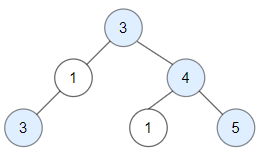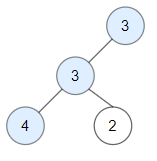## 题目

### A. 连续字符

• 1 <= s.length <= 500
• s 只包含小写英文字母。

### B. 最简分数

• 1 <= n <= 100

### C. 统计二叉树中好节点的数目

「好节点」X 定义为：从根到该节点 X 所经过的节点中，没有任何节点的值大于 X 的值。• 二叉树中节点数目范围是 [1, 10^5]
• 每个节点权值的范围是 [-10^4, 10^4]

### D. 数位成本和为目标值的最大数字

• 给当前结果添加一个数位（i + 1）的成本为 cost[i]cost 数组下标从 0 开始）。
• 总成本必须恰好等于 target
• 添加的数位中没有数字 0 。

• cost.length == 9
• 1 <= cost[i] <= 5000
• 1 <= target <= 5000